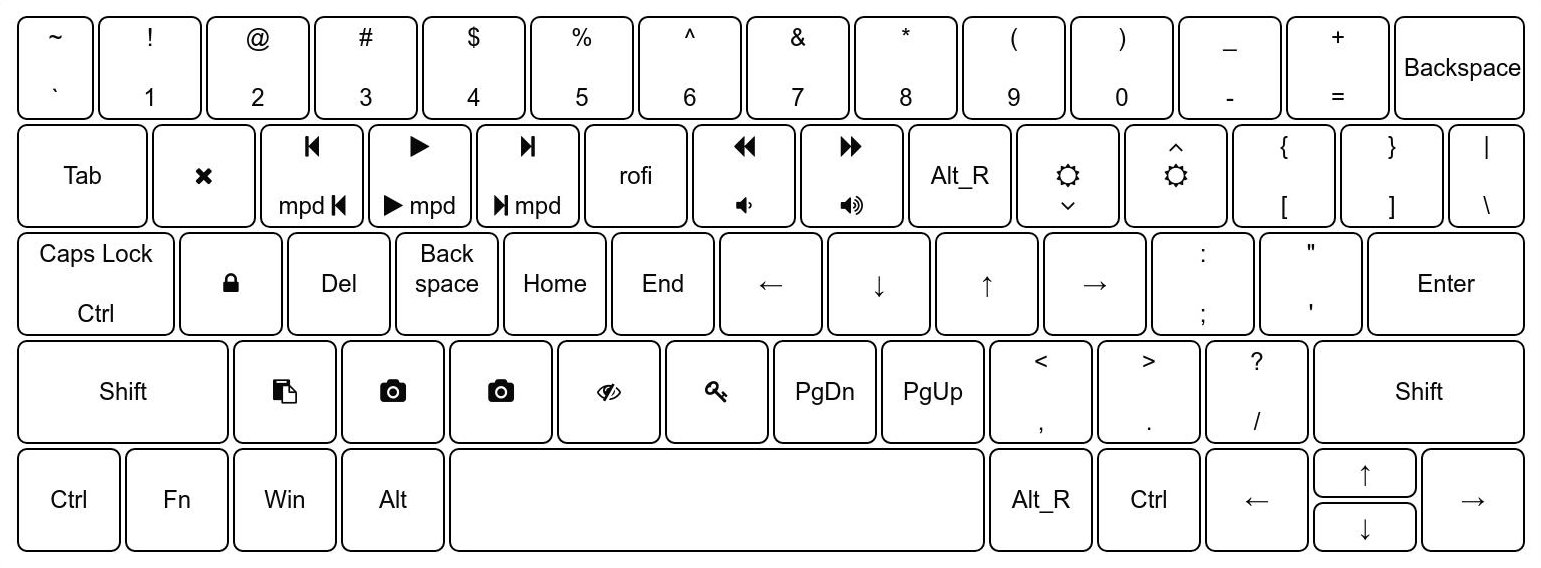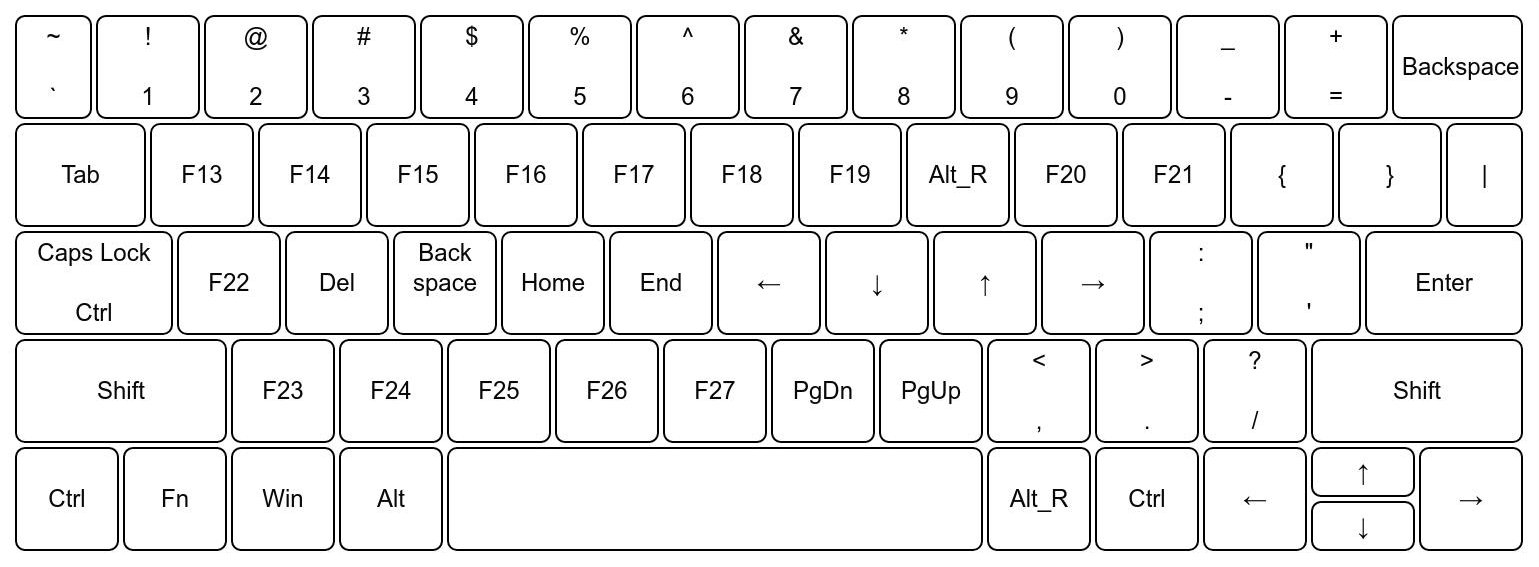# The Goal

## Insert Mode### Normal Mode#Inspired by vim, I wanted to create a layer on top of my keyboard which worked like a shortcut layer. So, to start off, I found out about XKB1. XKB is the Xorg Keyboard Extension which tells Xorg on how to react to input from keyboard. After reading through some source code, I found out that Xorg has support for function keys F1 - F352. The general idea here was:

• Create an insert mode layout for text input.
• Replace keys with relevant keys in normal mode (e.g. replace j with Down) and for keys that require executing a command, replace then with a function key above F12 (e.g. replace q with F13).
• Bind all the function keys above F12 to the respective functions.

To start off, I began a fresh Xorg session with nothing modifying the keys (removed `xmodmap` from startup) and first dumped the current layout into a file.

``````xkbcomp \$DISPLAY ~/.xkb/insert.xkb
``````

This was my starting point. I made changes to this file which were common to both Insert and Normal mode. e.g. replaced `Caps Lock` with `Ctrl` and made `Shift+Caps Lock` `Caps Lock`. Also, I unbound `Alt_R` as a modifier so that I could use that as a switch between Normal and Insert Mode.

Here is a diff between the original layout and Insert mode.

``````1323c1321
<     key <CAPS> {         [       Caps_Lock ] };
---
>     key <CAPS> {         [       Control_L,       Caps_Lock ] };
1551c1549
<     modifier_map Lock { <CAPS> };
---
>     modifier_map Control { <CAPS> };
1555d1552
<     modifier_map Mod1 { <RALT> };
``````

Next, I copied `~/.xkb/insert.xkb` to `~/.xkb/normal.xkb`. I replaced keys as per the plan.

Here is a diff between Insert mode and Normal mode.

``````1200c1200
<         symbols[Group1]= [               q,               Q ]
---
>         symbols[Group1]= [F13]
1204c1204
<         symbols[Group1]= [               w,               W ]
---
>         symbols[Group1]= [F14]
1208c1208
<         symbols[Group1]= [               e,               E ]
---
>         symbols[Group1]= [F15]
1212c1212
<         symbols[Group1]= [               r,               R ]
---
>         symbols[Group1]= [F16]
1216c1216
<         symbols[Group1]= [               t,               T ]
---
>         symbols[Group1]= [F17]
1220c1220
<         symbols[Group1]= [               y,               Y ]
---
>         symbols[Group1]= [F18]
1224c1224
<         symbols[Group1]= [               u,               U ]
---
>         symbols[Group1]= [F19]
1228c1228
<         symbols[Group1]= [               i,               I ]
---
>         symbols[Group1]= [Alt_R]
1232c1232
<         symbols[Group1]= [               o,               O ]
---
>         symbols[Group1]= [F20]
1236c1236
<         symbols[Group1]= [               p,               P ]
---
>         symbols[Group1]= [F21]
1244c1244
<         symbols[Group1]= [               a,               A ]
---
>         symbols[Group1]= [F22]
1248c1248
<         symbols[Group1]= [               s,               S ]
---
>         symbols[Group1]= [Delete]
1252c1252
<         symbols[Group1]= [               d,               D ]
---
>         symbols[Group1]= [BackSpace]
1256c1256
<         symbols[Group1]= [               f,               F ]
---
>         symbols[Group1]= [Home]
1260c1260
<         symbols[Group1]= [               g,               G ]
---
>         symbols[Group1]= [End]
1264c1264
<         symbols[Group1]= [               h,               H ]
---
>         symbols[Group1]= [Left]
1268c1268
<         symbols[Group1]= [               j,               J ]
---
>         symbols[Group1]= [Down]
1272c1272
<         symbols[Group1]= [               k,               K ]
---
>         symbols[Group1]= [Up]
1276c1276
<         symbols[Group1]= [               l,               L ]
---
>         symbols[Group1]= [Right]
1285c1285
<         symbols[Group1]= [               z,               Z ]
---
>         symbols[Group1]= [F23]
1289c1289
<         symbols[Group1]= [               x,               X ]
---
>         symbols[Group1]= [F24]
1293c1293
<         symbols[Group1]= [               c,               C ]
---
>         symbols[Group1]= [F25]
1297c1297
<         symbols[Group1]= [               v,               V ]
---
>         symbols[Group1]= [F26]
1301c1301
<         symbols[Group1]= [               b,               B ]
---
>         symbols[Group1]= [F27]
1305c1305
<         symbols[Group1]= [               n,               N ]
---
>         symbols[Group1]= [Next]
1309c1309
<         symbols[Group1]= [               m,               M ]
---
>         symbols[Group1]= [Prior]
``````

At this point, `normal.xkb` file defines the following layout.Now, we need a script that switches between layouts. To load an layout in Xorg, we use

``````xkbcomp ~/.xkb/normal.xkb "\$DISPLAY"
``````

Sway supports this via the input command in the following form.

``````swaymsg input '*' xkb_file ~/.xkb/normal.xkb
``````

The following script cycles through the layouts when it is called. It also allows to add more layouts later (just add them to layouts array and it will cycle in the order of the array).

``````#!/usr/bin/env bash
# Usage: xkb_swapper.sh [layout_name]

function set_layout() {
echo "Setting layout to \$1"
if [[ -v WAYLAND_DISPLAY ]]; then
swaymsg input '*' xkb_file ~/.xkb/"\$1".xkb
else
xkbcomp ~/.xkb/"\$1".xkb "\$DISPLAY"
fi
echo "\$1" > ~/.cache/xkb-curr-"\$DISPLAY"
}
layouts=(insert normal)
current_layout=\$(cat ~/.cache/xkb-curr-"\$DISPLAY" || echo "")

if [[ \$1 != "" ]]; then
set_layout "\$1"
exit
fi
if [[ \$current_layout == "" ]]; then
echo "No current layout found!"
set_layout "\${layouts}"
fi

i=0
while [[ \$i -lt \${#layouts[@]} ]]; do
if [[ \$current_layout == "\${layouts[\$i]}" ]]; then
new_idx="\$((i+1))"
if [[ \$new_idx -eq \${#layouts[@]} ]]; then
set_layout "\${layouts}"
else
set_layout "\${layouts[\$new_idx]}"
fi
exit
fi
((i++))
done

echo "Current Layout doesn't exist!"
set_layout "\${layouts}"
``````

The above script works with all Xorg based DE/WMs as well as Sway (wayland compositor). I saved it as `xkb_swapper.sh` in my `PATH`. Calling the script without any argument cycles through the layouts. If arguments are passed, the first argument is taken as layout name and layout is changed to that.

The last step is binding the function keys and `Alt_R` to commands to execute. Here are some of the parts of my i3 config that bind the function keys.

``````bindsym Alt_R exec xkb_swapper.sh
bindsym 0xffca kill
bindsym 0xffcf exec volchange -5
bindsym 0xffd0 exec volchange +5
bindsym 0xffd1 exec brightness -200
bindsym 0xffd2 exec brightness +200
bindsym 0xffcb exec mpc prev
bindsym 0xffcc exec mpc toggle
bindsym 0xffcd exec mpc next
``````

`i3` doesn’t seem to accept `F13` - `F35` as keynames however it accepts the keycodes2. Here is a small list for easy access.

``````0xffbe   F1
0xffbf   F2
0xffc0   F3
0xffc1   F4
0xffc2   F5
0xffc3   F6
0xffc4   F7
0xffc5   F8
0xffc6   F9
0xffc7   F10
0xffc8   F11
0xffc9   F12
0xffca   F13
0xffcb   F14
0xffcc   F15
0xffcd   F16
0xffce   F17
0xffcf   F18
0xffd0   F19
0xffd1   F20
0xffd2   F21
0xffd3   F22
0xffd4   F23
0xffd5   F24
0xffd6   F25
0xffd7   F26
0xffd8   F27
0xffd9   F28
0xffda   F29
0xffdb   F30
0xffdc   F31
0xffdd   F32
0xffde   F33
0xffdf   F34
0xffe0   F35
``````

# Bonus: Displaying the current mode in your bar

The script stores the mode in `~/.cache/xkb-curr-\$DISPLAY`. `cat` that and wrap in your bar’s config. Here is my config for i3status-rust .

``````[[block]]
block = "custom"
command = "echo -en '\\uf11c '; cat ~/.cache/xkb-curr-\$DISPLAY"
interval = 0.5
``````

1. As always, the Arch Wiki page on XKB is a nice place to start. ↩︎

2. You can find all the defined keys in `/usr/include/X11/keysymdef.h`↩︎